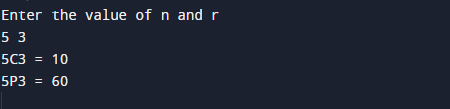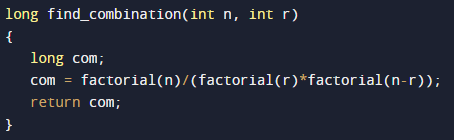C Program to find ncr and npr

In this tutorial you will learn about the C Program to find ncr and npr and its application with practical example.

In this tutorial, we will learn to create a C program that will Calculate Permutation(nPr) and Combination(nCr)  using C programming.

Prerequisites

Before starting with this tutorial we assume that you are best aware of the following C programming topics:

• C Operators.
• Functions in C.
• Factorial.
• C Recursion.

What Is Permutation (nPr) and Combination (nCr)?

Permutation:=>

permutation is an ordered arrangement . The term arrangement is referred if the order of things is considered.
Formula. nPr = n!/(n-r)!

Note->Permutation  shows arrange r things out of n.

What is Combination?

“Combination” is any selection or pairing of values within a single category
i.e  combination means selection of things, Order of objects does not matter.
Formula. n!/(r!*(n-r)!).

Note->Combination shows  to select r things out of n.

OutputIn the above program, we have first declared and initialized a set variables required in the program.Firstly we ask user to give the value of n,r by prompting user and by getting the value we pass the value or n and r to function find_combination(n,r)This function take two parameter while calling and within function we call another function named factorial that will find the possible combination of n and r and at the end this function returns total combination back to caller object and same process we will use for permutation.This function take two parameter while calling and within function we call another function named factorial that will find the possible permutation of n and r and at the end this function returns total permutation back to caller object and at end we will print total number of combination and permutation.

In this tutorial we have learn about the C Program to find ncr and npr and its application with practical example. I hope you will like this tutorial.